# How to☝️ Sort by Last Name in Google Sheets

To sort by last name in Google Sheets, select a blank cell, go to the Formula bar, enter the formula =SORT(A1:A5,INDEX(SPLIT(A1:A5,” “),,2),1), and hit Enter

Keep reading to find out more about this topic. Learn how to sort full names by last name in just a few seconds. Let’s explore it together!

## Method 1: The Quickest Way to Sort by Last Name

Take a look at the following step-by-step illustrated guide for a detailed explanation on how to sort a list of names by last name.

Difficulty: Beginner

Time Estimate: 7 seconds

1. Click on the cell where you want the alphabetized list.
2.  Navigate to the Formula bar and type the formula =SORT(A1:A5,INDEX(SPLIT(A1:A5,” “),,2),1), where A1:A5 is the cell range with your data.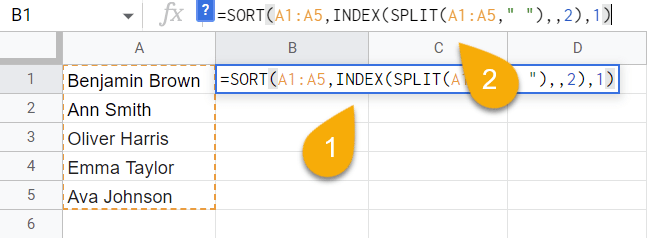1. Press the Enter key on your keyboard.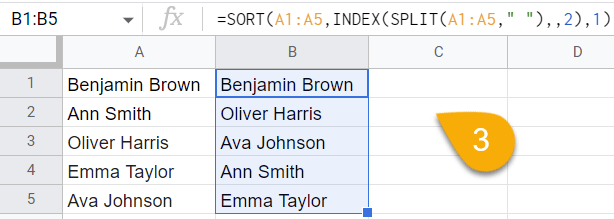Easy as ABC! The list has now been sorted according to last name.

## Method 2: Using the REGEX Methods

As an alternative method to alphabetize full names by the last name in Google Sheets, you can also use the one described below to help sort them quickly and easily.

Difficulty: Beginner

Time Estimate: 10 seconds

1. Select the blank cell where you want the result.
2. Go to the Formula bar and enter the formula =INDEX(SORT(REGEXEXTRACT(A1:A5,”((.*)( .*))”),3,1),,1). In this case, A1:A5 is the range of cells with your data.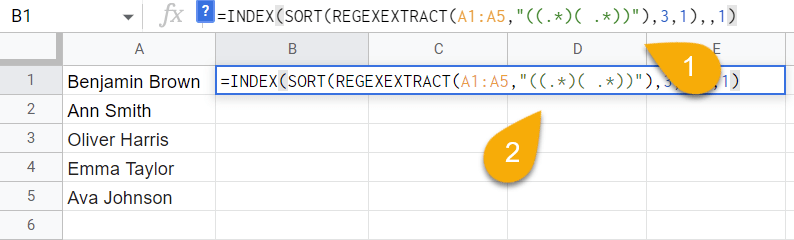1. Hit the Enter key to get the result.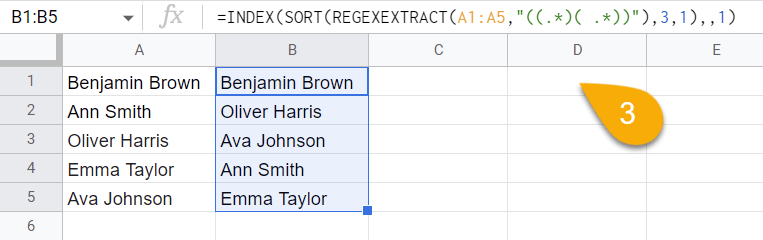Voila! Once again, the names have been sorted.

There is another formula that uses the REGEX function to obtain the same outcome:

1. Click on a blank cell.
2. Navigate to the Formula bar and type the formula =SORT(A1:A5,REGEXEXTRACT(A1:A5,”(?: )(\w*)”),1), where A1:A5 is your cell range with names.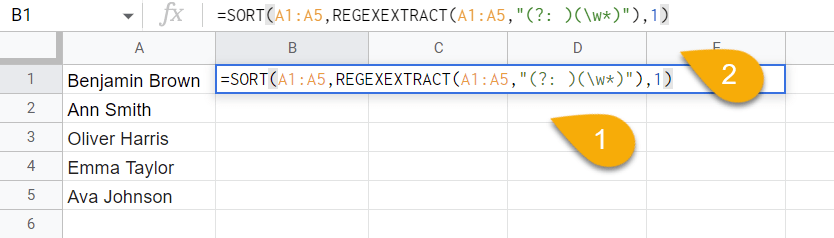1. Lastly, press Enter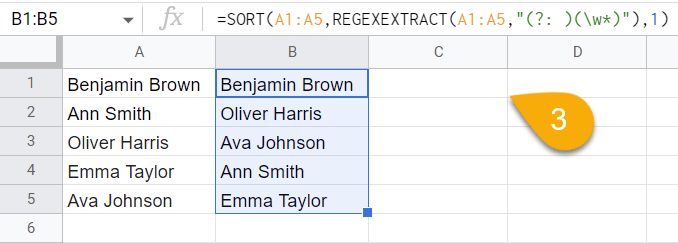Easy as pie!

## How to Sort the Last Name Separately in Google Sheets

If you want to take the last names only and sort them in alphabetical order on their own, simply do the following:

Difficulty: Beginner

Time Estimate: 15 seconds

1. Choose the blank cell where you want your list.
2. In the Formula bar, enter the formula RIGHT(A1,LEN(A1)-FIND(“<^>”,SUBSTITUTE(A1,” “,”<^>”,LEN(\$A\$1)-LEN(SUBSTITUTE(\$A\$1,” “,””))),1)), where A1 is the cell with a full name.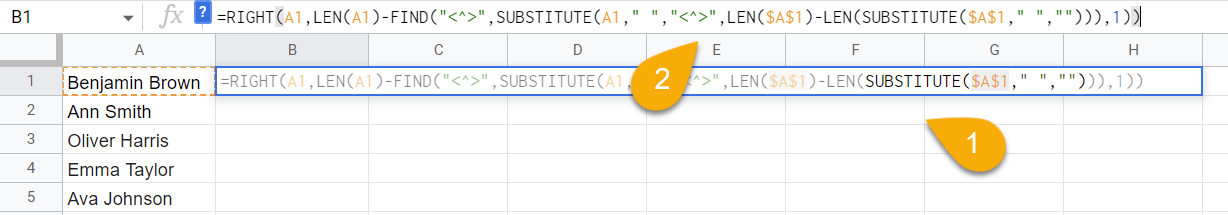1. Hit the Enter key on the keyboard.
2. Drag the cell with the result downward from the lower right corner icon to copy the formula through the rest of the list. This pulls just the last name from the cells.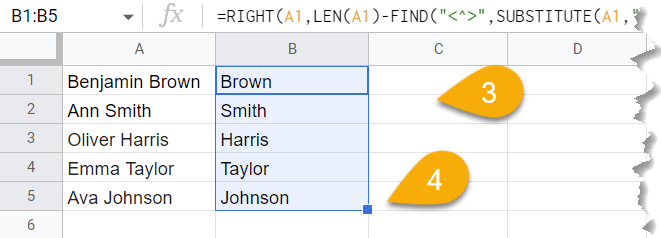1. Go to the Data menu.
2. Select the Sort range option.
3. Pick the Sort range by column B (A to Z) or (Z to A) order.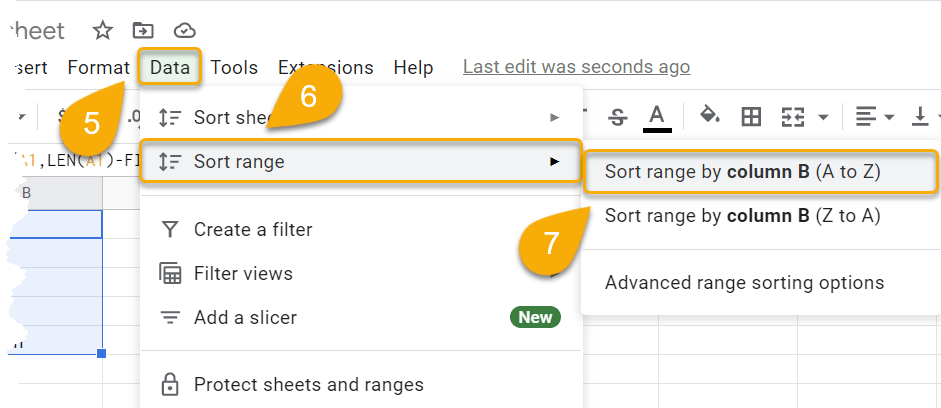Easy-peasy! That’s all it takes.